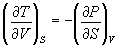5HC - Chemical Engineering Thermodynamics - April 2000

1. What is a state function? Give examples
2. Explain enthalpy
3. What is an isolated system?
4. Define specific heat capacity
5. Explain isothermal process
6. Is entropy of the universe changing? Explain
7. What is fugacity
8. Explain Gibbs free energy
9. Explain zeroth law of thermodynamics
10. Define activity coefficient
11. What is Henry's law?
12. What is the criteria of equilibrium in terms of chemical potential?
13. Explain virial equations
14. State the properties of refrigerants
15. What is Joule Thomson effect?
16. Define heat of solution
17. What is Margules equation?
18. Define theoretical flame temperature
19. What is equilibrium?
20. Define critical temperature and critical pressure
21.

22. (a) Define and give examples to the following terms: (4 x 2 = 8)

(i) Isochoric process

(ii) Source

(iii) System

(iv) Surroundings

(b) Discuss with an example the concept of a reversible process. (4)

23. Or

24. 220 kg of CO2 gas at 27oC and 1 atmosphere is compressed adiabatically to 1/5th of its volume. It is then cooled to its original temperature. Find Q, DU, and work done for each step and for the entire process.
25. Discuss ideal refrigeration cycle and show that C.O.P of a reversible refrigerating machine is independent of the fluid used.
26. Or

27. (a) Show that in multistage compressor, the interstage pressure is the geometric mean of the initial and final pressures for the minimum work. (6)

(b) Hydrogen is to be compressed in four stages from 1 to 211 kgf/cm2. What should be the pressure between the satges? (6)

28. Derive the following: (2 x 6 = 12)
29. (a)(b) ( U/ V) = - [P + T ( V/ T)P / ( V/ P)T]

Or
30. Derive from the first principles the following relations:

(a) dU = TdS - PdV

(b) dH = TdS + VdP

(c) dA = -SdT - PdV

(d) dG = -SdT + VdP

31. Discuss in detail various thermodynamic diagrams and their usefulness.
32. Or

33. Given the Van Laar constants A = -0.8 and B = 1.2, find the composition of the vapor phase of a binary system whose liquid phase composition is 50 mole %. Vapor pressure of components 1 and 2 are 680 and 710 mm Hg respectively.
34. Acetic acid is esterified as per the reactions

CH3COOH(l) + C2H5OH(l) à CH3COOC2H5(l) + H2O(l) at 100oC

Given: DHo298 = 13100 J
DFo298 = 9270 J

Assuming DHo is a constant, find equilibrium constant at 100oC. What is the composition at 100oC?

35. Or
36. Discuss the effect of temperature, pressure and inerts on equilibrium constant.